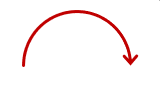# How to draw curve with arc?

• Hi All,
I'm sharing the code, Using this code drawing half circle(curve ) how to add arrow at the end of this curve ?
Or is there other way to draw curve with direction/arrow. but I'm drawing this curve inside the circle so i think using radius its better way.
CHPlotCurve--derived from QwtPlotCurve class
CHPlotGraph2D-2D Graph derived from QwtPlot

``````  for (int irows = 0, maxI = table1->rowCount(); irows < maxI; ++irows)
{
double x1 = table1->data(table1->index(irows, 1)).toDouble(&ok1);
double y1 = table1->data(table1->index(irows, 2)).toDouble(&ok2);
QVector <double> ax,ay;
for (double angle = 0; angle <= 3.2; angle += 0.00314)
{
double ax1 = x1 + (r / 2) * cos(angle);
double ay1 = y1 + (r / 2) * sin(angle);
ax.append(ax1);
ay.append(ay1);
CHPlotCurve* arc = (CHPlotCurve*)pGr->insertCurve("arc", CHPlotGraph2D::Line, false);
arc->setSamples(ax, ay);
arc->setPen(Qt::blue);
}
``````

Thankyou. Your answers are welcome

• How to draw like this ?